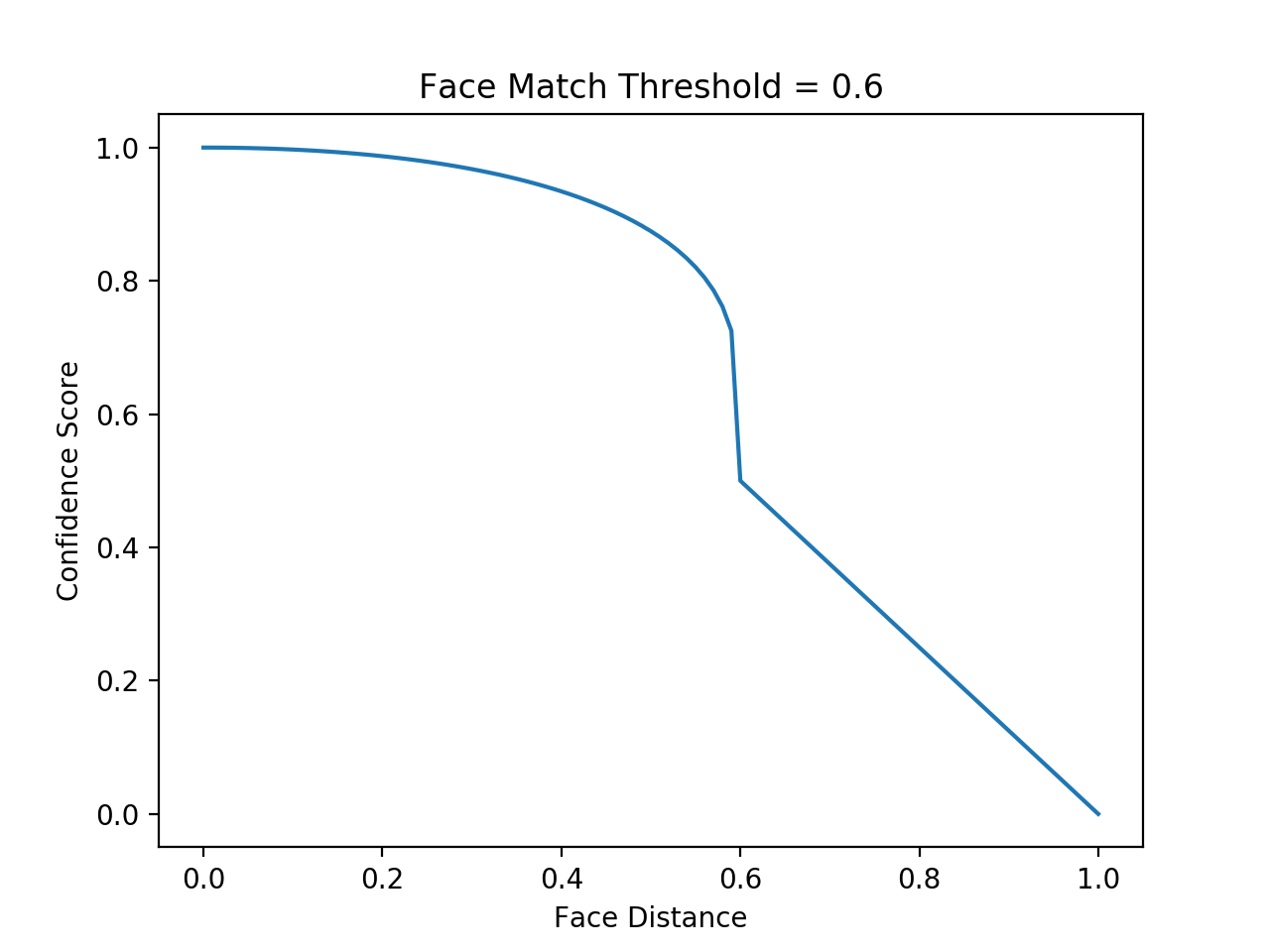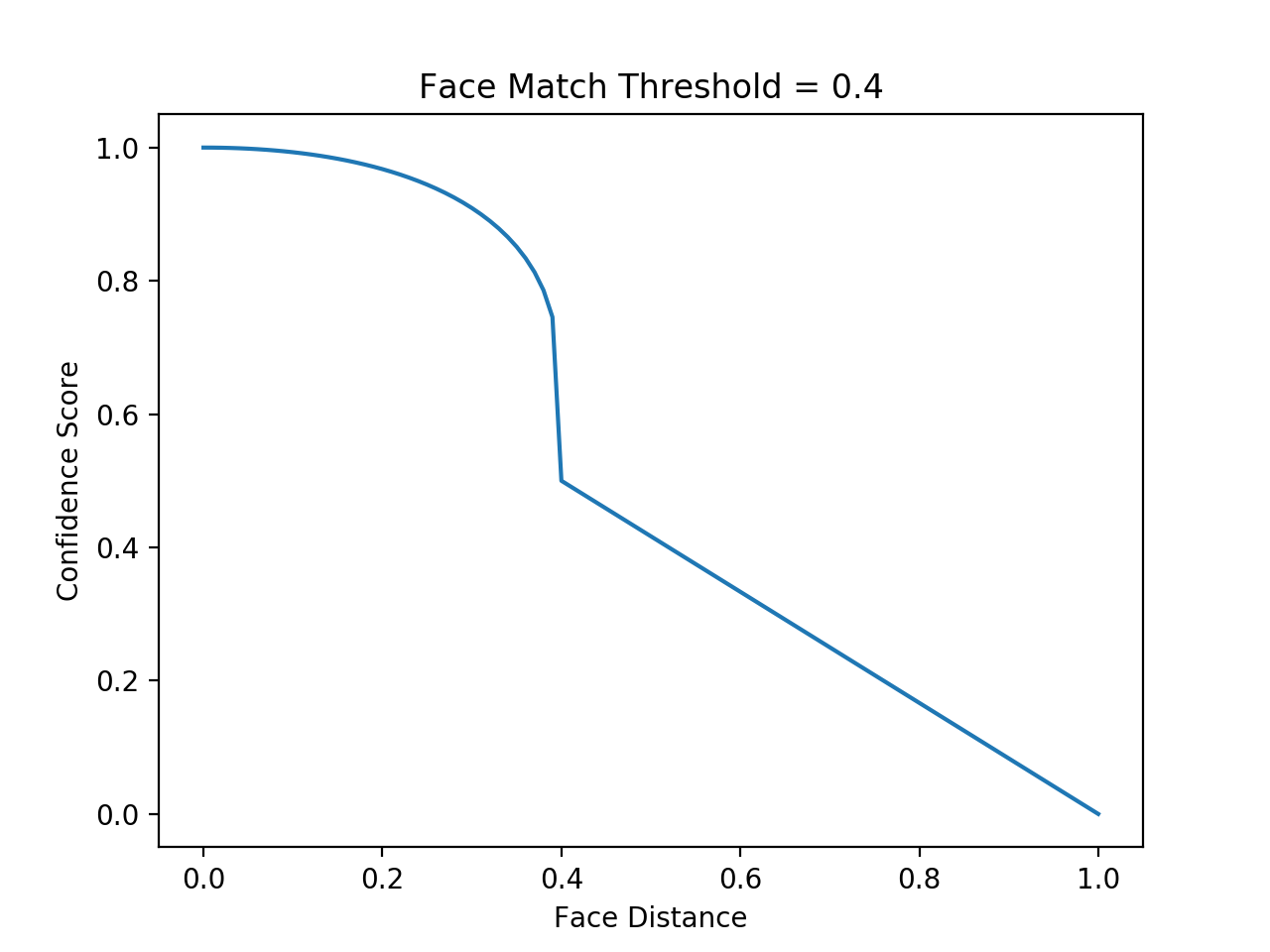{{ message }}

# Calculating Accuracy as a Percentage

##### Clone this wiki locally

This library represents each face as a point in an imaginary 128-dimensional space. To check if two faces match, it checks if the distance between those two points is less than 0.6 (the default threshold). Using a lower threshold than 0.6 makes the face comparison more strict.

But in some cases, people want to display a "percent match" between to faces instead of the face distance, which will be an arbitrary number like 0.4718.

In that case, you need to convert a face distance score into a percent match score. There's no "right answer" of how to do this without building a test set and evaluating some results to assign a confidence score, but here's one possible answer that "feels right" in many cases:

```import math

def face_distance_to_conf(face_distance, face_match_threshold=0.6):
if face_distance > face_match_threshold:
range = (1.0 - face_match_threshold)
linear_val = (1.0 - face_distance) / (range * 2.0)
return linear_val
else:
range = face_match_threshold
linear_val = 1.0 - (face_distance / (range * 2.0))
return linear_val + ((1.0 - linear_val) * math.pow((linear_val - 0.5) * 2, 0.2))```

With a face match threshold of 0.6, the result looks like this:And if you set a threshold of 0.4, it looks like this: